Type
Quiz
Book Title
Fundamentals of Corporate Finance Standard Edition 9th Edition
ISBN 13
978-0073382395

### 978-0073382395 Chapter 10 Questions and Problems 16-29

April 3, 2019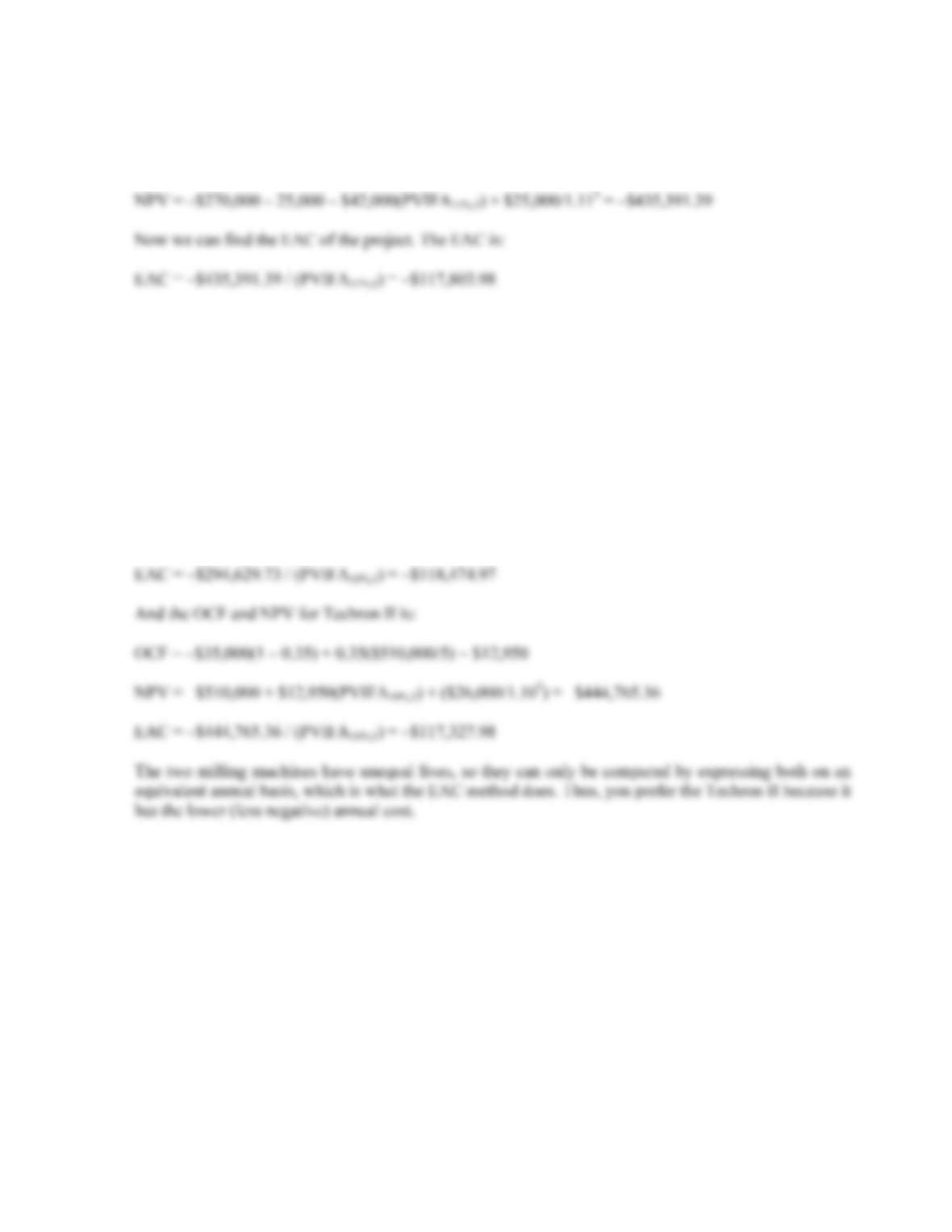CHAPTER 10 B-185
16. To calculate the EAC of the project, we first need the NPV of the project. Notice that we include the
NWC expenditure at the beginning of the project, and recover the NWC at the end of the project. The
NPV of the project is:
17. We will need the aftertax salvage value of the equipment to compute the EAC. Even though the
equipment for each product has a different initial cost, both have the same salvage value. The aftertax
salvage value for both is:
Both cases: aftertax salvage value = \$40,000(1 – 0.35) = \$26,000
To calculate the EAC, we first need the OCF and NPV of each option. The OCF and NPV for Techron I
is:
OCF = –\$67,000(1 – 0.35) + 0.35(\$290,000/3) = –9,716.67
NPV = –\$290,000 – \$9,716.67(PVIFA10%,3) + (\$26,000/1.103) = –\$294,629.73
18. To find the bid price, we need to calculate all other cash flows for the project, and then solve for the bid
price. The aftertax salvage value of the equipment is:
Aftertax salvage value = \$70,000(1 – 0.35) = \$45,500
Now we can solve for the necessary OCF that will give the project a zero NPV. The equation for the
NPV of the project is:
NPV = 0 = –\$940,000 – 75,000 + OCF(PVIFA12%,5) + [(\$75,000 + 45,500) / 1.125]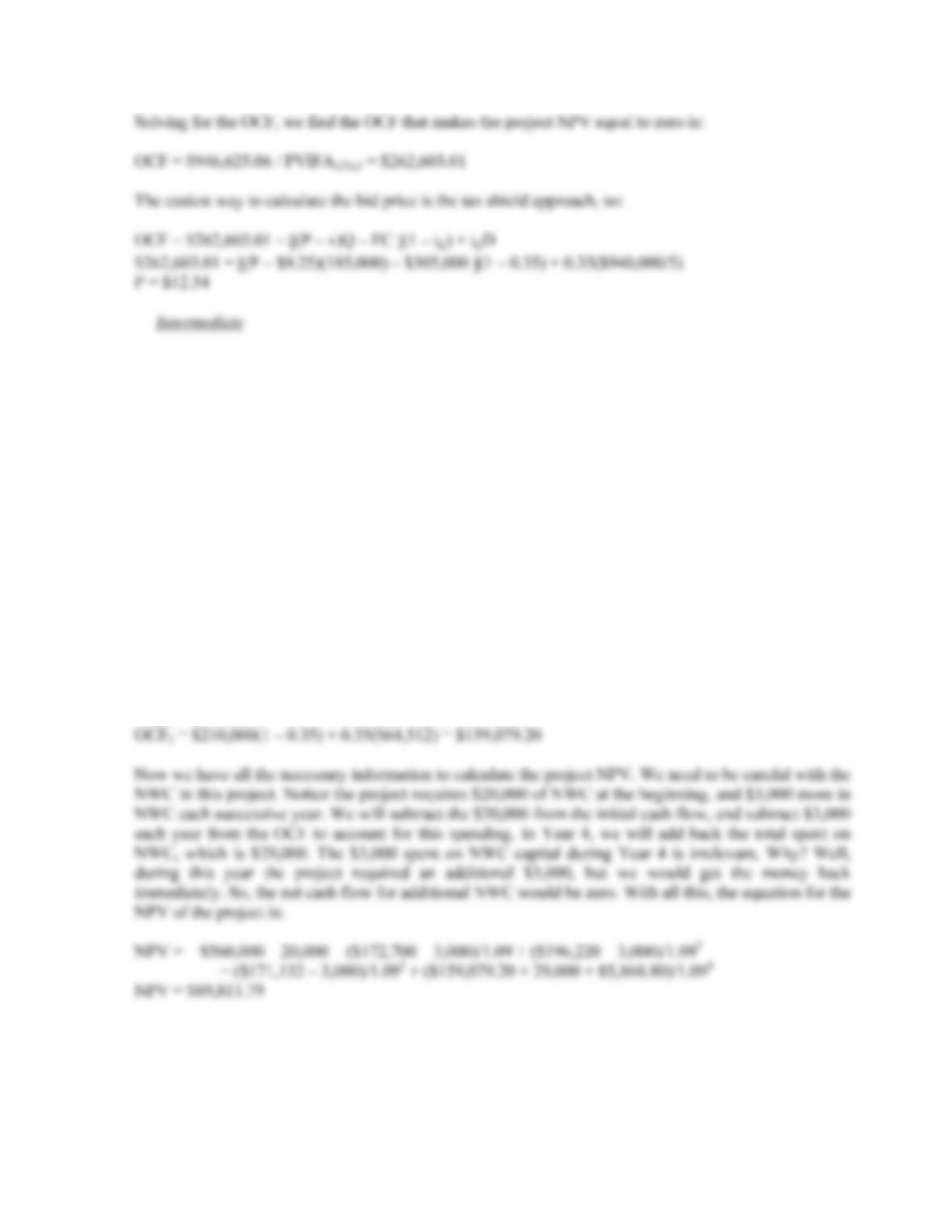B-186 SOLUTIONS
19. First, we will calculate the depreciation each year, which will be:
D
1 = \$560,000(0.2000) = \$112,000
D
2 = \$560,000(0.3200) = \$179,200
D
3 = \$560,000(0.1920) = \$107,520
D
4 = \$560,000(0.1152) = \$64,512
The book value of the equipment at the end of the project is:
BV4 = \$560,000 – (\$112,000 + 179,200 + 107,520 + 64,512) = \$96,768
The asset is sold at a loss to book value, so this creates a tax refund.
After-tax salvage value = \$80,000 + (\$96,768 – 80,000)(0.35) = \$85,868.80
So, the OCF for each year will be:
OCF1 = \$210,000(1 – 0.35) + 0.35(\$112,000) = \$172,700
OCF2 = \$210,000(1 – 0.35) + 0.35(\$179,200) = \$196,220
OCF3 = \$210,000(1 – 0.35) + 0.35(\$107,520) = \$171,132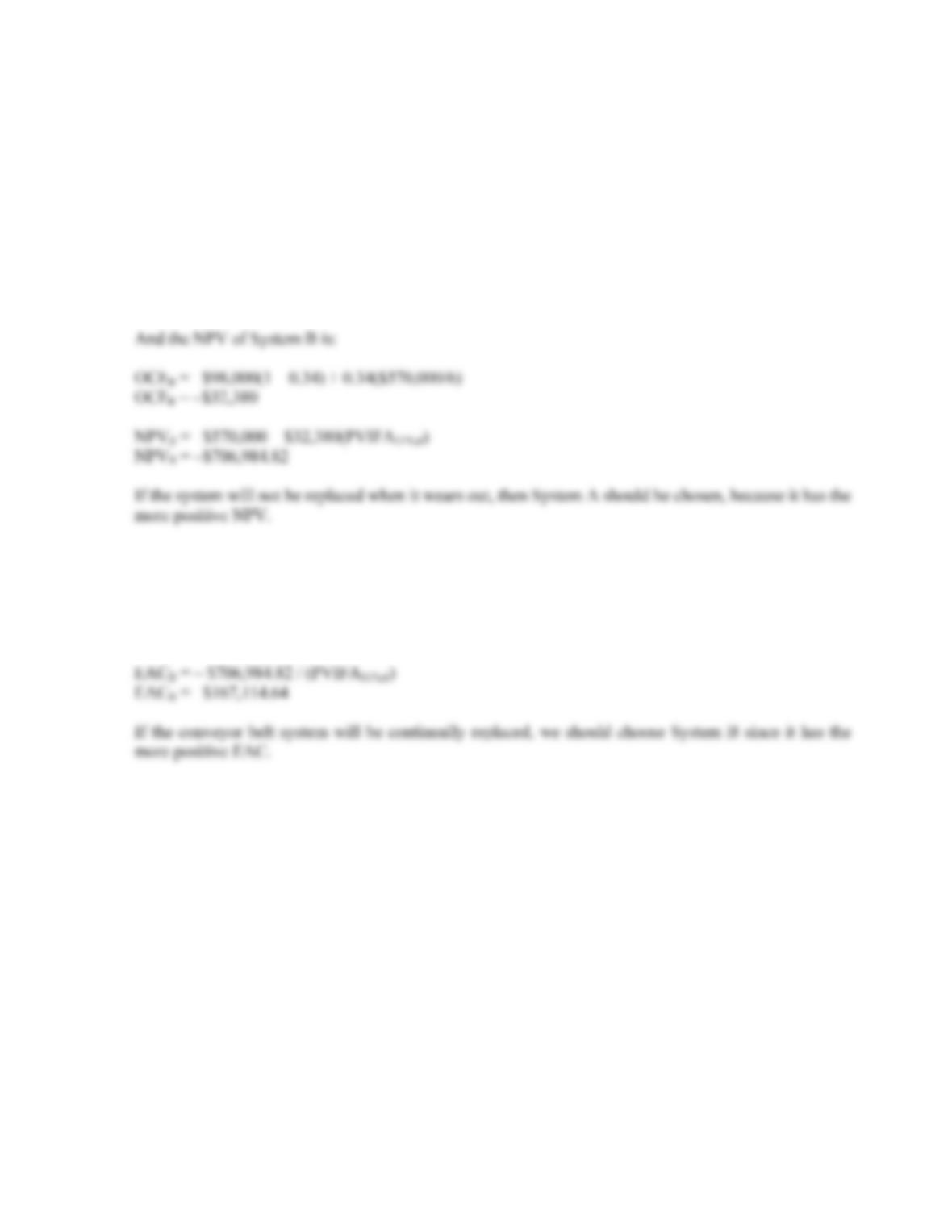CHAPTER 10 B-187
20. If we are trying to decide between two projects that will not be replaced when they wear out, the proper
capital budgeting method to use is NPV. Both projects only have costs associated with them, not sales,
so we will use these to calculate the NPV of each project. Using the tax shield approach to calculate the
OCF, the NPV of System A is:
OCFA = –\$110,000(1 – 0.34) + 0.34(\$430,000/4)
OCFA = –\$36,050
NPVA = –\$430,000 – \$36,050(PVIFA11%,4)
NPVA = –\$541,843.17
21. If the equipment will be replaced at the end of its useful life, the correct capital budgeting technique is
EAC. Using the NPVs we calculated in the previous problem, the EAC for each system is:
EACA = –\$541,843.17 / (PVIFA11%,4)
EACA = –\$174,650.33
22. To find the bid price, we need to calculate all other cash flows for the project, and then solve for the bid
price. The aftertax salvage value of the equipment is:
After-tax salvage value = \$540,000(1 – 0.34)
After-tax salvage value = \$356,400
Now we can solve for the necessary OCF that will give the project a zero NPV. The current aftertax
value of the land is an opportunity cost, but we also need to include the aftertax value of the land in five
years since we can sell the land at that time. The equation for the NPV of the project is:
NPV = 0 = –\$4,100,000 – 2,700,000 – 600,000 + OCF(PVIFA12%,5) – \$50,000(PVIFA12%,4)
+ {(\$356,400 + 600,000 + 4(50,000) + 3,200,000] / 1.125}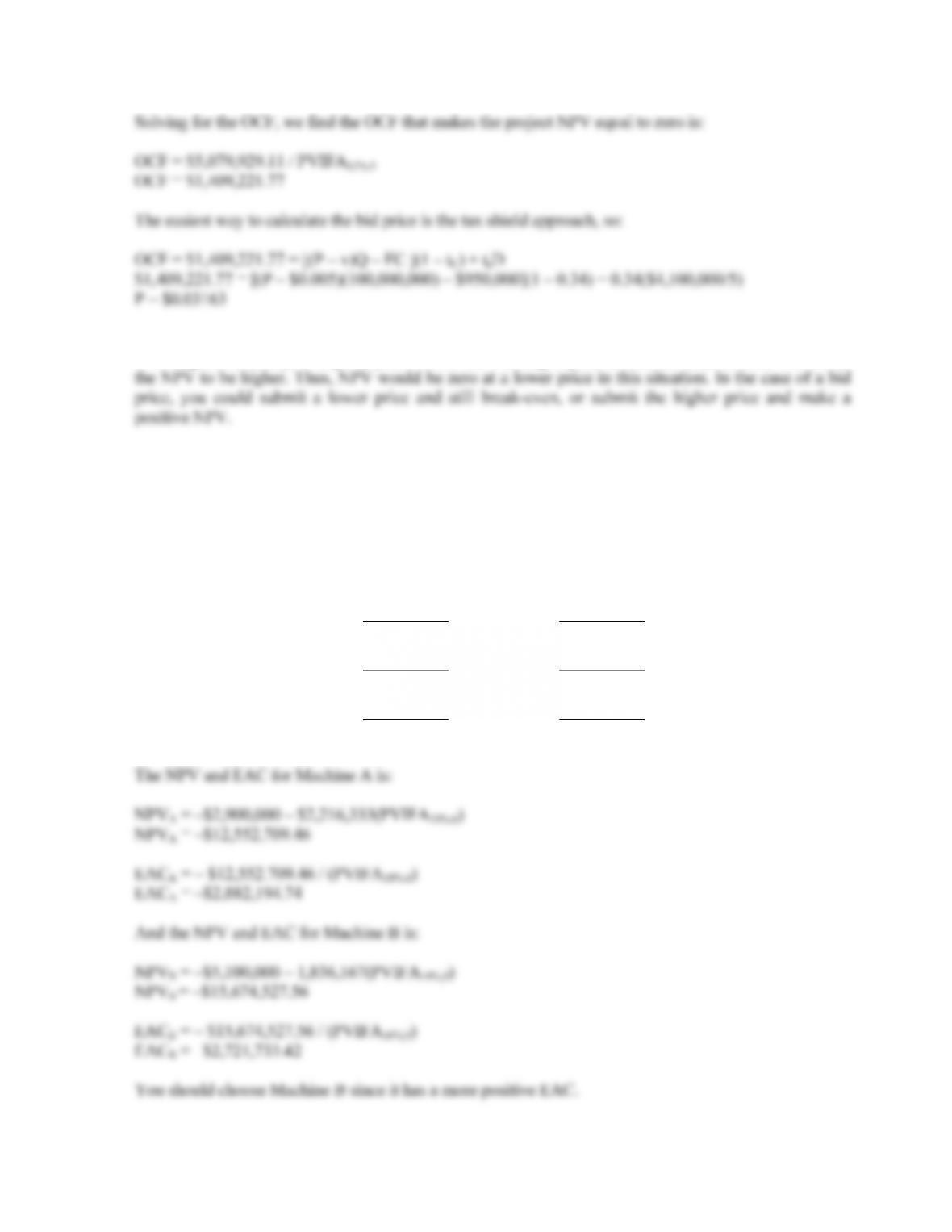B-188 SOLUTIONS
23. At a given price, taking accelerated depreciation compared to straight-line depreciation causes the NPV
to be higher; similarly, at a given price, lower net working capital investment requirements will cause
24. Since we need to calculate the EAC for each machine, sales are irrelevant. EAC only uses the costs of
operating the equipment, not the sales. Using the bottom up approach, or net income plus depreciation,
method to calculate OCF, we get:
Machine A Machine B
Variable costs –\$3,500,000 –\$3,000,000
Fixed costs –170,000 –130,000
Depreciation –483,333 –566,667
EBT –\$4,153,333 –\$3,696,667
Tax 1,453,667 1,293,833
Net income –\$2,699,667 –\$2,402,833
+ Depreciation 483,333 566,667
OCF –\$2,216,333 –\$1,836,167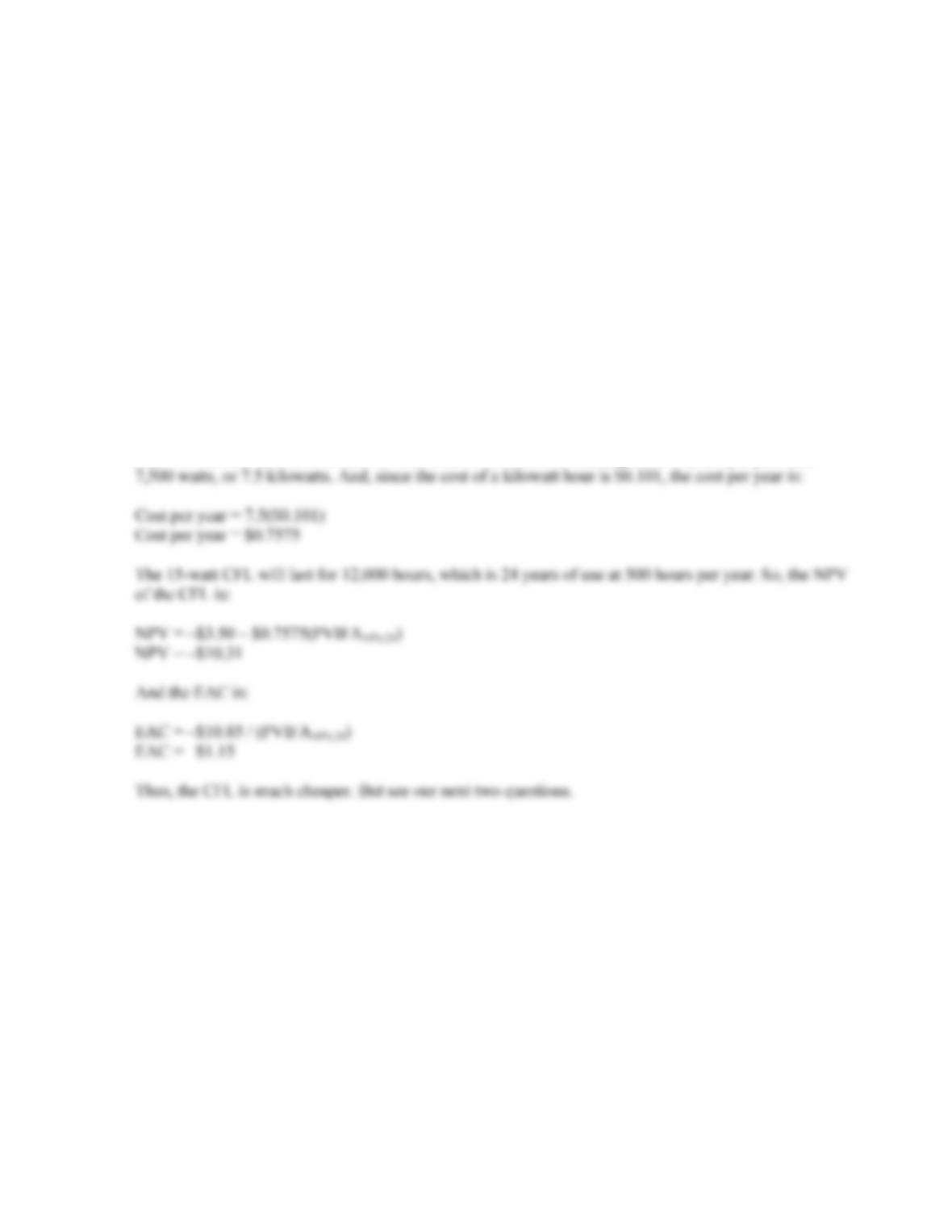CHAPTER 10 B-189
25. A kilowatt hour is 1,000 watts for 1 hour. A 60-watt bulb burning for 500 hours per year uses
30,000 watt hours, or 30 kilowatt hours. Since the cost of a kilowatt hour is \$0.101, the cost per year is:
Cost per year = 30(\$0.101)
Cost per year = \$3.03
The 60-watt bulb will last for 1,000 hours, which is 2 years of use at 500 hours per year. So, the NPV of
the 60-watt bulb is:
NPV = –\$0.50 – \$3.03(PVIFA10%,2)
NPV = –\$5.76
And the EAC is:
EAC = –\$5.83 / (PVIFA10%,2)
EAC = –\$3.32
Now we can find the EAC for the 15-watt CFL. A 15-watt bulb burning for 500 hours per year uses
26. To solve the EAC algebraically for each bulb, we can set up the variables as follows:
W = light bulb wattage
C = cost per kilowatt hour
H = hours burned per year
P = price the light bulb
The number of watts use by the bulb per hour is:
WPH = W / 1,000
And the kilowatt hours used per year is:
KPY = WPH × H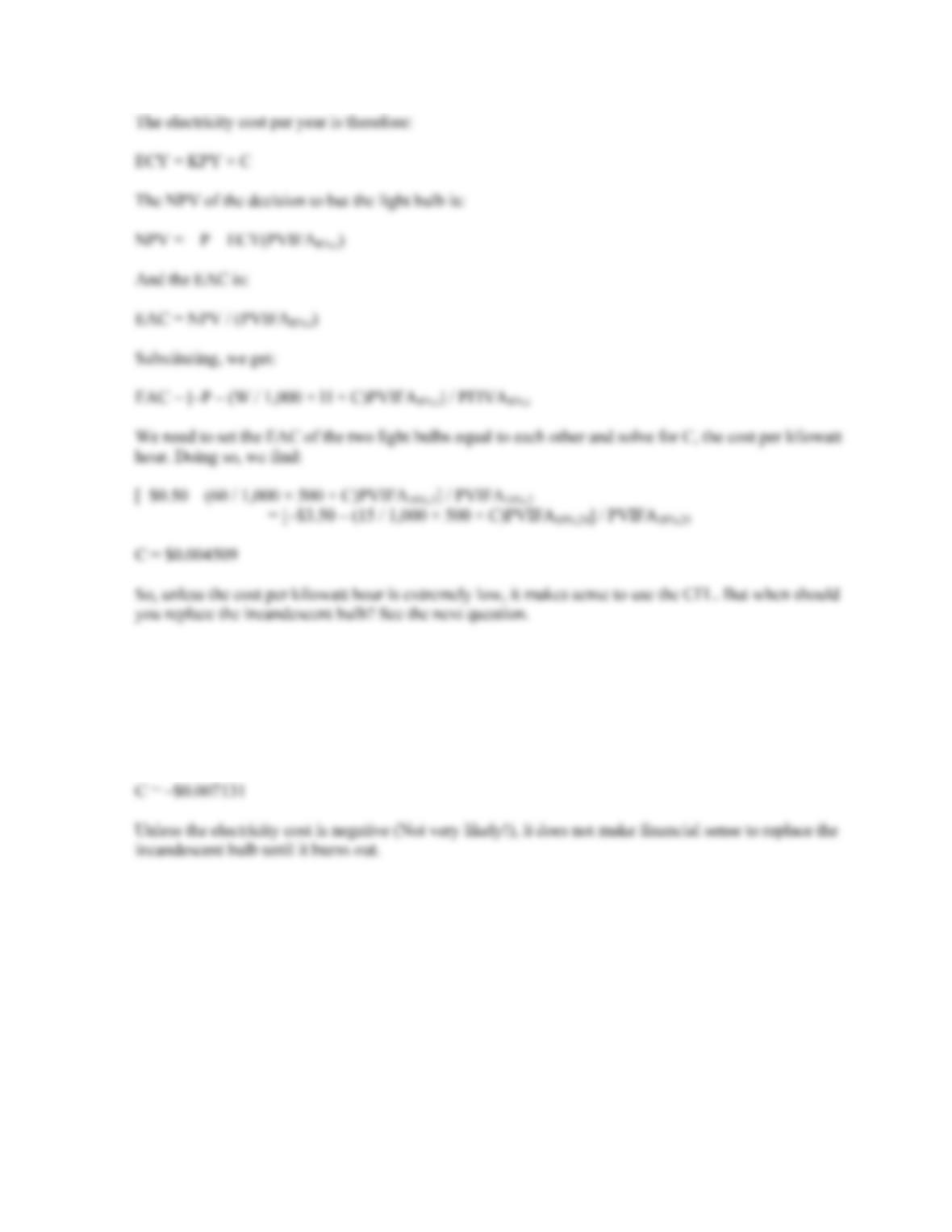B-190 SOLUTIONS
27. We are again solving for the breakeven kilowatt hour cost, but now the incandescent bulb has only 500
hours of useful life. In this case, the incandescent bulb has only one year of life left. The breakeven
electricity cost under these circumstances is:
[–\$0.50 – (60 / 1,000 × 500 × C)PVIFA10%,1] / PVIFA10%,1
= [–\$3.50 – (15 / 1,000 × 500 × C)PVIFA10%,24] / PVIFA10%,24
28. The debate between incandescent bulbs and CFLs is not just a financial debate, but an environmental one
as well. The numbers below correspond to the numbered items in the question:
1. The extra heat generated by an incandescent bulb is waste, but not necessarily in a heated
structure, especially in northern climates.
2. Since CFLs last so long, from a financial viewpoint, it might make sense to wait if prices are
declining.
3. Because of the nontrivial health and disposal issues, CFLs are not as attractive as our previous
analysis suggests.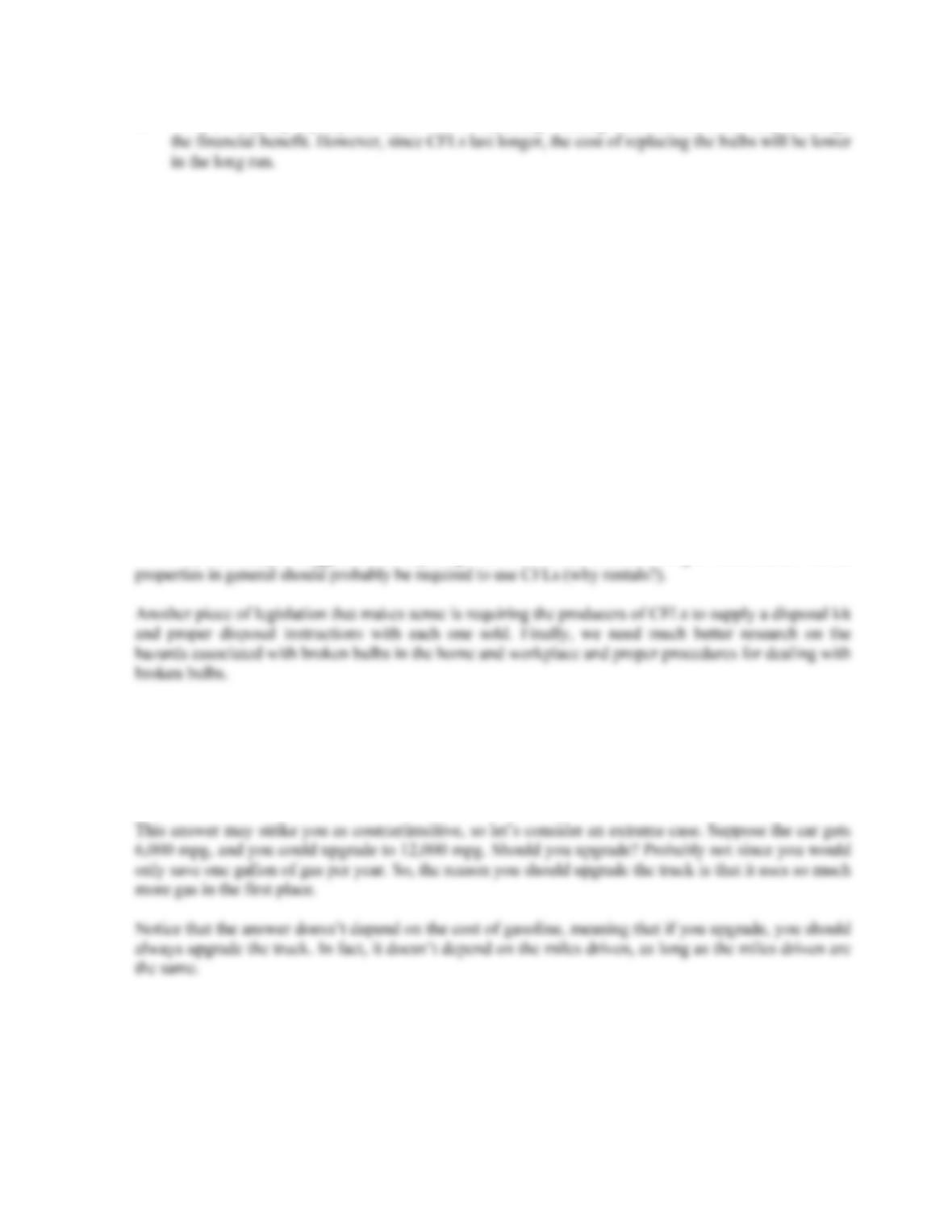CHAPTER 10 B-191
4. From a company’s perspective, the cost of replacing working incandescent bulbs may outweigh
5. Because incandescent bulbs use more power, more coal has to be burned, which generates more
mercury in the environment, potentially offsetting the mercury concern with CFLs.
6. As in the previous question, if CO2 production is an environmental concern, the the lower power
consumption from CFLs is a benefit.
7. CFLs require more energy to make, potentially offsetting (at least partially) the energy savings
from their use. Worker safety and site contamination are also negatives for CFLs.
8. This fact favors the incandescent bulb because the purchasers will only receive part of the benefit
from the CFL.
9. This fact favors waiting for new technology.
10. This fact also favors waiting for new technology.
While there is always a “best” answer, this question shows that the analysis of the “best” answer is not
always easy and may not be possible because of incomplete data. As for how to better legislate the use
of CFLs, our analysis suggests that requiring them in new construction might make sense. Rental
29. Surprise! You should definitely upgrade the truck. Here’s why. At 10 mpg, the truck burns 12,000 / 10
= 1,200 gallons of gas per year. The new truck will burn 12,000 / 12.5 = 960 gallons of gas per year, a
savings of 240 gallons per year. The car burns 12,000 / 25 = 480 gallons of gas per year, while the new
car will burn 12,000 / 40 = 300 gallons of gas per year, a savings of 180 gallons per year, so it’s not
even close.